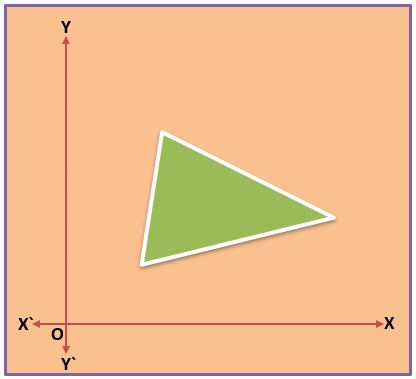## Area of Triangle in Coordinate Geometry Class 10th

Introduction Before finding the Area of a Triangle in Coordinate Geometry, We know the area of a triangle if its Base and Height are given Area of triangle = ½⨯Base⨯Height If all three sides of a triangle are given then the area of the triangle by Heron’s formula Area of triangle = √s(s-a)(s-b)(s-c)                     Where, s …